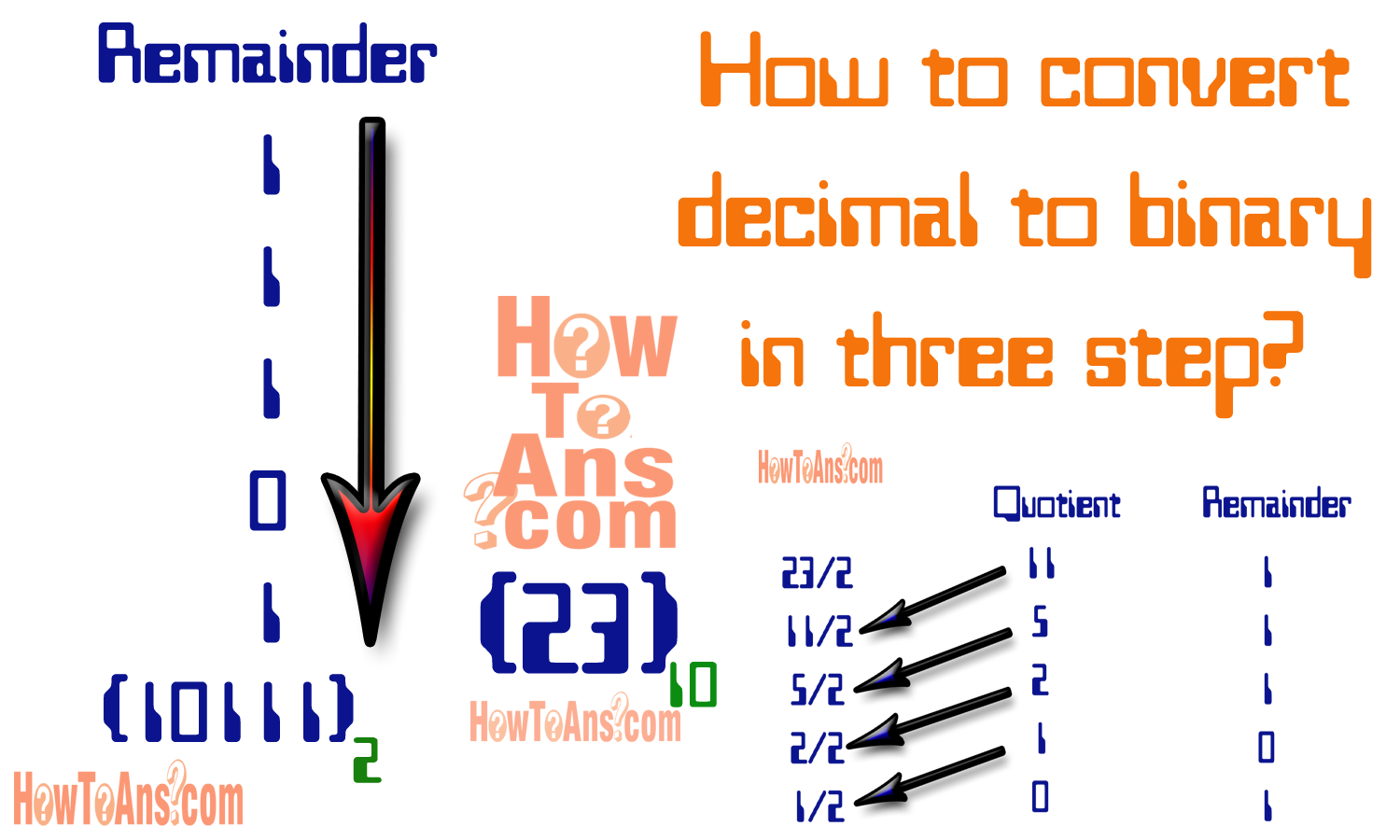# How to convert decimal to binary in three easy step

## How to convert decimal to binary in three easy step?

How-to-convert-decimal-to-binaryHow-to-convert-decimal-to-binary?
Today, we are telling you How to convert decimal to binary? The base of binary is two (2) that are '0' and '1'. And the base of decimal is ten (10) that is from 0 to 9. Any decimal number can be converted into binary number. In the binary table, the value of 0 to 9 are define in binary form. From this table we can see the value in decimal form from (0 to 9) and also we can see the value in binary form from (0 to 9). That is showing below:-
But if we want to convert more than 9 value then we not see it into binary table. So, today we are telling you how to convert decimal to binary? in three step. We are define These three step with the help of example. But before these three step we are telling you How to convert decimal to binary? in short form that are given below:-

Conversion of integer can be obtained by continuously dividing by two (2) and separated the remainder whereas in the fraction parts, the conversion is done by multiplying by two (2) and keeping track of integer generating.

### Take decimal number (First step):-

In this step we take a decimal number which is convert into binary value that is greater than 9. For example we take 23 decimal number that is convert into binary value.

### Divided by two (2) and separate the remainder (Second step):-

In this step, we will telling you that how to divide by two and separate the remainder. We take a value 23 that is convert into binary value.
firstly, we divide the decimal value by 2 and after that we write the quotient and remainder i.e 0 and 1. Till, we will divide continuously until the quotient is not 0. First we divide 23 by 2 and we write the quotient and remainder. After that we will use quotient as a divider continuously until the quotient is zero.

### Write remainder bottom to top (Third step):-

We have remainder i.e write from bottom to top when we write answer. Many time we write the remainder from top to bottom when write answer but it is wrong. By this our right result will made wrong. So please keep it in mind that result are write  from bottom to top.

YEeEhhhooooooooooooooooo! We have done!
It was very important for computer learner and many one continually asking from us that how to convert decimal-to-binary? now we have discussed that how you can do that and keep it in mind for future and after today don't ask any one even let know others.So keep reading and learning because you are with How to Ans -Don't worry about how to do?
Yes one thing also keep in mind that if you are getting problem in doing that please ask us in the box given below or if you fully understood that don't forget to appreciate us...